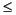External InterfacesData Types in MATLAB

Complex Double-Precision Matrices

The most common data type in MATLAB is the complex double-precision, nonsparse matrix. These matrices are of type `double` and have dimensions `m`-by-`n`, where `m` is the number of rows and `n` is the number of columns. The data is stored as two vectors of double-precision numbers - one contains the real data and one contains the imaginary data. The pointers to this data are referred to as `pr` (pointer to real data) and `pi` (pointer to imaginary data), respectively. A real-only, double-precision matrix is one whose `pi` is `NULL`.

Numeric Matrices

MATLAB also supports other types of numeric matrices. These are single-precision floating-point and 8-, 16-, and 32-bit integers, both signed and unsigned. The data is stored in two vectors in the same manner as double-precision matrices.

Logical Matrices

The logical data type represents a logical `true` or `false` state using the numbers `1` and `0`, respectively. Certain MATLAB functions and operators return logical `1` or logical `0` to indicate whether a certain condition was found to be true or not. For example, the statement `(5` `*` `10)` `>` `40` returns a logical 1 value.

MATLAB Strings

MATLAB strings are of type `char` and are stored the same way as unsigned 16-bit integers except there is no imaginary data component. Unlike C, MATLAB strings are not null terminated.

Cell Arrays

Cell arrays are a collection of MATLAB arrays where each `mxArray` is referred to as a cell. This allows MATLAB arrays of different types to be stored together. Cell arrays are stored in a similar manner to numeric matrices, except the data portion contains a single vector of pointers to `mxArrays`. Members of this vector are called cells. Each cell can be of any supported data type, even another cell array.

Structures

A 1-by-1 structure is stored in the same manner as a 1-by-n cell array where `n` is the number of fields in the structure. Members of the data vector are called fields. Each field is associated with a name stored in the `mxArray`.

Objects

Objects are stored and accessed the same way as structures. In MATLAB, objects are named structures with registered methods. Outside MATLAB, an object is a structure that contains storage for an additional classname that identifies the name of the object.

Multidimensional Arrays

MATLAB arrays of any type can be multidimensional. A vector of integers is stored where each element is the size of the corresponding dimension. The storage of the data is the same as matrices.

Empty Arrays

MATLAB arrays of any type can be empty. An empty `mxArray` is one with at least one dimension equal to zero. For example, a double-precision `mxArray` of type `double`, where `m` and `n` equal 0 and `pr` is `NULL`, is an empty array.

Sparse Matrices

Sparse matrices have a different storage convention than full matrices in MATLAB. The parameters `pr` and `pi` are still arrays of double-precision numbers, but there are three additional parameters, `nzmax`, `ir`, and `jc`:

• `nzmax` is an integer that contains the length of `ir`, `pr`, and, if it exists, `pi`. It is the maximum possible number of nonzero elements in the sparse matrix.
• `ir` points to an integer array of length `nzmax` containing the row indices of the corresponding elements in `pr` and `pi`.
• `jc` points to an integer array of length `N+1` that contains column index information. For `j`, in the range `0 ``j ``N-1`, `jc[j]` is the index in `ir` and `pr` (and `pi` if it exists) of the first nonzero entry in the `j`th column and
`jc[j+1] - 1` index of the last nonzero entry. As a result, `jc[N]` is also equal to `nnz`, the number of nonzero entries in the matrix. If `nnz` is less than `nzmax`, then more nonzero entries can be inserted in the array without allocating additional storage.Data Storage Using Data Types